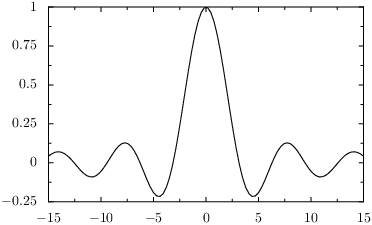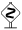# PyX — Example: graphs/function.py

0.2 KB
48.4 KB
50.8 KB
25.4 KB
31.3 KB

## Plotting a function graph```from pyx import *

g = graph.graphxy(width=8)
g.plot(graph.data.function("y(x)=sin(x)/x", min=-15, max=15))
g.writeEPSfile("function")
g.writePDFfile("function")
g.writeSVGfile("function")
```

### Description

Here, we explain how to plot the graph of a real-valued mathematical function of a single real parameter.

As in the previous example, we first create a `graph.graphxy` instance passing the width as argument. We then pass a `graph.data.function` instance to the `plot` method of the graph. As first argument, we pass the function in the form "y(x) = f(x)". Here, the left-hand side of the equation defines which parameter is independent (here: `x`) and which one is dependent (here: `y`). We also need to inform PyX about the range of the independent variable. This is done by passing the parameters `min` and `max`.In order to increase the resolution of the function graph, you can use the parameter `points` of the `graph.data.function` class to increase the number of sampling points from its default value of `100`.

Note that the default graph style for function data is `graph.style.line` since PyX assumes a continuous x-range.You only need to pass the `min` and `max` parameters to the `graph.data.function` class, if PyX cannot figure out an axis range by itself. Thus, an alternative way to achieve the above result would look like

```g = graph.graphxy(width=8, x=graph.axis.linear(min=-15, max=15))
g.plot(graph.data.function("y(x)=sin(x)/x"))
```

Here, we explicitely define an x-axis range by passing an appropriate argument to the `x` parameter of the `graph.graphxy` class.For PyX it does not matter, whether you plot a function of a parameter `x` or a parameter `y`. Thus, you may as well write

```g.plot(graph.data.function("x(y)=sin(y)/y", min=-15, max=15))
```

to obtain a plot where the y-coordinate is the independent one.